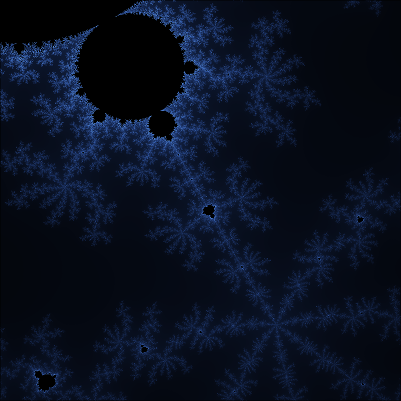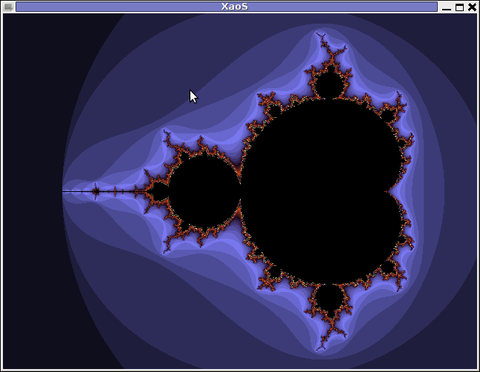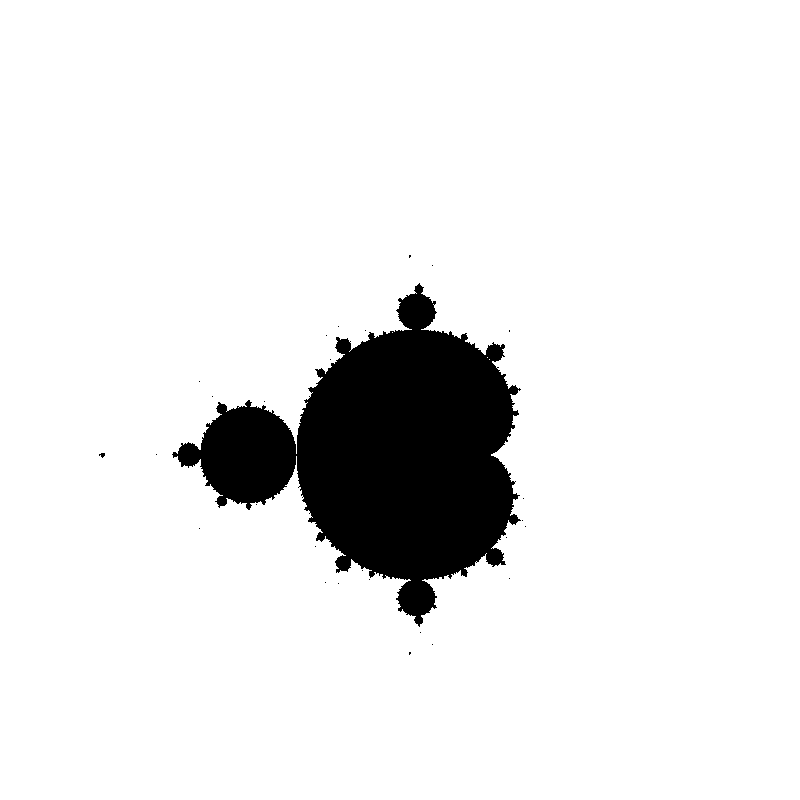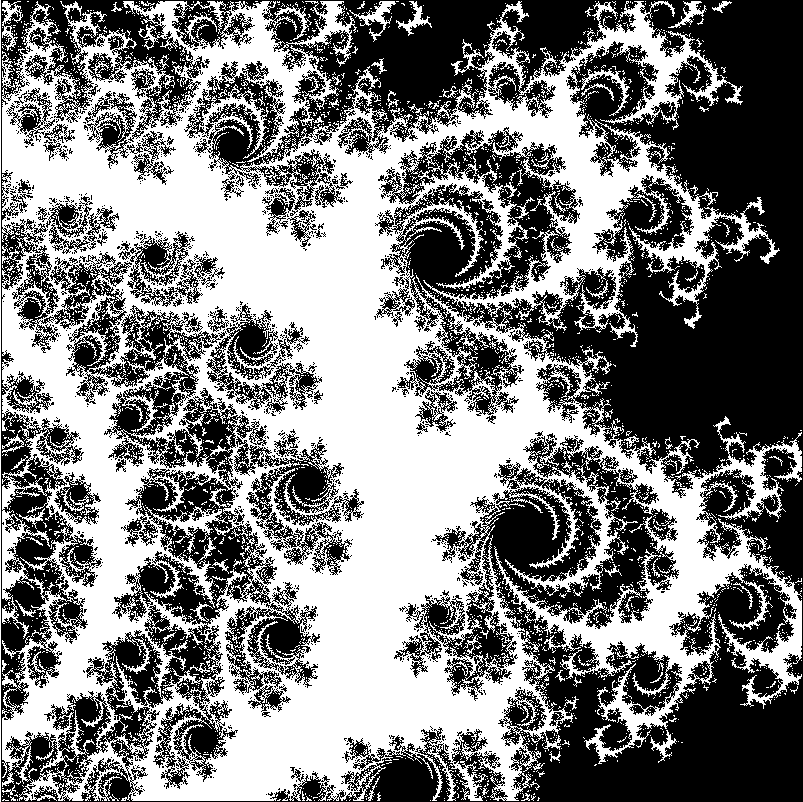# Rendering the Mandelbrot Set With WebGLDo you like this picture? Want to know how to generate images like this? Then read on! Or, if you already know how to do it, you can have some fun playing with the end result.

## The Mandelbrot Set

The Mandelbrot set is a mathematical object that has a special place in my heart. My first encounter with it was in high school, when I tried Linux for the first time. A friend showed me a distro called Knoppix, which you could run without actually installing it on your machine. It came with lots of pre-packaged software. Among it was XaoS, a program for exploring fractals.It's still as glorious now as it was back then.

It was mind-shattering, a true demonstration of the beauty of mathematics. I'd boot into Linux just to play with XaoS.

Recently, I was watching some videos from Numberphile, and one of them brought me back to the past:

It occurred to me that it should be quite easy to write a fast Mandelbrot renderer using a GLSL pixel shader. So I did that, and here's how it works.

## Some Theory

Numberphile's video does an excellent job explaining what the Mandelbrot set is, but I'll do it here again for the sake of completeness (plus, it never hurts to see the same thing explained in two ways).

I'm going to assume that you are already familiar with complex numbers and how their addition and multiplication works. If you're not (or you just need to refresh your memory), go read the wikipedia article, it's fairly simple.

The Mandelbrot set is defined as follows. Let fc(z) = z2 + c (where c is an arbitrary complex number) be a function on the domain of complex numbers. Consider a sequence where the first element is fc(0), and each consecutive element is derived by applying fc to the preceding element:

z0 = fc(0)
z1 = fc(z0)
z2 = fc(z1)
...
zn = fc(zn)
...

A complex number c belongs in the Mandelbrot set if the corresponding sequence of absolute values |z0|, |z1|, ... is bounded. In other words, c is in the Mandelbrot set if there exists a value s such that, for any i, |zi| ≤ s.

## Writing it In Code

This definition is pretty simple, but how would one write a program to check if a complex number belongs to the Mandelbrot set? It's not possible to do with exact precision (we can't exactly check all the elements of zn for all possible values of s), but we can get a very good approximation. First, there's this handy fact that if any member of zn becomes greater than 2 in absolute value, then the sequence is definitely unbounded. And if we only check the first hundred or so elements of the sequence, it turns out to be a pretty good approximation as long as you don't look too closely (checking more elements will yield more precise results but will also slow things down). Simply put, to decide if c is in the set, we just need to check the that the first few elements of the sequence are less than or equal to 2. The more elements we check the more is the probability that our decision is correct.

Let's write some GLSL code!

``````
/* Fragment shader that renders Mandelbrot set */
precision mediump float;

/* Width and height of screen in pixels */
uniform vec2 u_resolution;

/* Point on the complex plane that will be mapped to the center of the screen */
uniform vec2 u_zoomCenter;

/* Distance between left and right edges of the screen. This essentially specifies
which points on the plane are mapped to left and right edges of the screen.
Together, u_zoomCenter and u_zoomSize determine which piece of the complex
plane is displayed. */
uniform float u_zoomSize;

/* How many iterations to do before deciding that a point is in the set. */
uniform int u_maxIterations;

vec2 f(vec2 z, vec2 c) {
return mat2(z,-z.y,z.z)*z + c;
}

void main() {
vec2 uv = gl_FragCoord.xy / u_resolution;

/* Decide which point on the complex plane this fragment corresponds to.*/
vec2 c = u_zoomCenter + (uv * 4.0 - vec2(2.0)) * (u_zoomSize / 4.0);

/* Now iterate the function. */
vec2 z = vec2(0.0);
bool escaped = false;
for (int i = 0; i < 10000; i++) {
/* Unfortunately, GLES 2 doesn't allow non-constant expressions in loop
conditions so we have to do this ugly thing instead. */
if (i > u_maxIterations) break;
z = f(z, c);
if (length(z) > 2.0) {
escaped = true;
break;
}
}
gl_FragColor = escaped ? vec4(1.0) : vec4(vec3(0.0), 1.0);
}
```
```

The result looks like this:Two-colored image of the Mandelbrot setSame image, zoomed in

## Where Do the Colors Come From?

But this looks pretty boring, doesn't it? Let's add some variety then. Usually, each point is assigned a color based on how many iterations it took to detect that it doesn't belong to the set. A very straightforward approach is to divide the actual number of iterations over the maximum number of iterations. This will give us a value between 0 and 1, which can be used directly to produce a grayscale image:

``````
/* Fragment shader that renders Mandelbrot set */
precision mediump float;

...

void main() {
...
int iterations;
for (int i = 0; i < 10000; i++) {
/* Unfortunately, GLES 2 doesn't allow non-constant expressions in loop
conditions so we have to do this ugly thing instead. */
if (i > u_maxIterations) break;
iterations = i;
z = f(z, c);
if (length(z) > 2.0) {
escaped = true;
break;
}
}
gl_FragColor = escaped ? vec4(vec3(float(iterations)) / float(u_maxIterations), 1.0) : vec4(vec3(0.0), 1.0);
}
```
```Grayscale image of the set, zoomed in.

But of course we can do better. One easy method would be to use a n x 1 pixel strip with smooth color transitions as a texture, and sample the final color from it, using iterations/maxIterations as the texture coordinate. However, you don't really need to use a texture, you can just generate a color by interpolating between a few colors:

``````
/* Linearly interpolate between the four given colors. */
vec3 palette(float t, vec3 c1, vec3 c2, vec3 c3, vec3 c4) {
float x = 1.0 / 3.0;
if (t < x) return mix(c1, c2, t/x);
else if (t < 2.0 * x) return mix(c2, c3, (t - x)/x);
else if (t < 3.0 * x) return mix(c3, c4, (t - 2.0*x)/x);
return c4;
}

void main() {
...
int iterations;
for (int i = 0; i < 10000; i++) {
/* Unfortunately, GLES 2 doesn't allow non-constant expressions in loop
conditions so we have to do this ugly thing instead. */
if (i > u_maxIterations) break;
iterations = i;
z = f(z, c);
if (length(z) > 2.0) {
escaped = true;
break;
}
}
gl_FragColor = escaped ? vec4(palette(float(iterations)/ float(u_maxIterations), vec3(0.02, 0.02, 0.03), vec3(0.1, 0.2, 0.3), vec3(0.0, 0.3, 0.2), vec3(0.0, 0.5, 0.8)), 1.0) : vec4(vec3(0.3, 0.5, 0.8), 1.0);
}
```
```Using a greenish-blue palette.

We're pretty much there, but the palette function might be too branchy for your tastes. In that case, there's a trick that allows generating nice smooth palettes with just a single cosine computation and some additions/multiplications (all credit goes to Inigo Quilez, read his post for an explanation of how it works). This one is a little bit harder to control though (note that the vectors you pass into the function are NOT the colors you're going to be getting!)

``````
vec3 palette(float t, vec3 a, vec3 b, vec3 c, vec3 d) {
return a + b*cos( 6.28318*(c*t+d) );
}
```
```I like this coloring the most.

That's it! You can play around with the final version.

## Note on Zoom Depth

Unfortunately, the magic breaks down if you zoom in far enough, but that's just the limitation of the machine: there are only so many bits in a floating point number.

I do have an idea of how to achieve "infinite" zoom: if you render the picture in software, and use an arbitrary-precision floating point library like GNU MP, you should be able to zoom in a lot more. The drawback is that it would be exteremely slow compared to the OpenGL-based version, although you could combine the two methods: use hardware-accelerated rendering for normal zoom levels and software rendering when high precision is required (not to mention, you can parallelize the software implementation as well as speed it up with SIMD instructions).

Like this post? Follow this blog on Twitter for more!